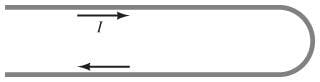# Magnetic field at the center of a semi-circle

## Homework StatementFind B at the center of the 3.2-cm-radius semicircle in the figure(Figure 1) . The straight wires extend a great distance outward to the left and carry a current I=6.4A.

## Homework Equations

(Magnetic field for a current carrying loop)
$\overrightarrow{B}=\frac{\mu_0I}{2R}$

## The Attempt at a Solution

• Assuming that the two straight segments create magnetic fields that just cancel each other out, since their currents run opposite of each other.
• Assuming the unshown segment somewhere far off to the left has insignificant influence (I think that's what "extend a great distance outward to the left" implies)

So I'm left with a semi-circle of radius .032 m and I need to find the magnetic field at the center.
Logically the field will just be half of that of a full circle, so:

$\overrightarrow{B_\frac{1}{2}}=\frac{1}{2}\frac{\mu_0I}{2R}=\frac{\mu_0I}{4R}=\frac{8.8542\cdot 10^{-12}\cdot 6.4}{4\cdot 0.032}=4.4271\cdot 10^{-10}$

Rounds to 4.4E-10 (Mastering Physics asks for two significant figures)

Program marks it as incorrect. I'm not sure where to go from here. I considered magnetic moment, but aside from halving the magnetic moment, I'm not sure what I could do.

TSny
Homework Helper
Gold Member
Hello vanillaatbest. Welcome to PF!

Assuming that the two straight segments create magnetic fields that just cancel each other out, since their currents run opposite of each other.

You'll need to think about this more carefully.Remember the right hand rule for determining the direction of magnetic field produced by a current element.

TSny
Homework Helper
Gold Member
Is this correct?

I'm not sure. It looks to me like the bottom part of your figure is correct, but the top part is not. But that could be that my brain is interpreting the 3D nature of the picture differently than you intended (like an optical illusion).In the attached figure, consider the blue and green sections of current.

Can you state the direction of the magnetic field at the red dot due to the blue section? (In or out of the page?)

Similarly, for the green section.

#### Attachments

Thanks for helping TSny,
I believe the magnetic field of the blue section at the red dot would be coming out of the screen.

The green section would be into the screen.

TSny
Homework Helper
Gold Member
Thanks for helping TSny,
I believe the magnetic field of the blue section at the red dot would be coming out of the screen.

Hmm. Take a look here and see if that makes you want to change your answer.

...oh god

I've been using my left hand

TSny
Homework Helper
Gold Member
Ah. A common slip.

•1 person
Ok. So in this case both the green and blue section would generate mag fields that point INTO the screen.

The semi-circle would generate a mag field that points OUT of the screen.

Wait nope. Used my left hand again. The semi-circle's mag field would also point INTO the screen.

Thank you for your help TSny. I'm going to take a break. If I find a solution later, I'll post it.